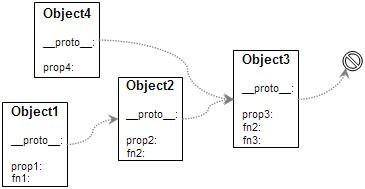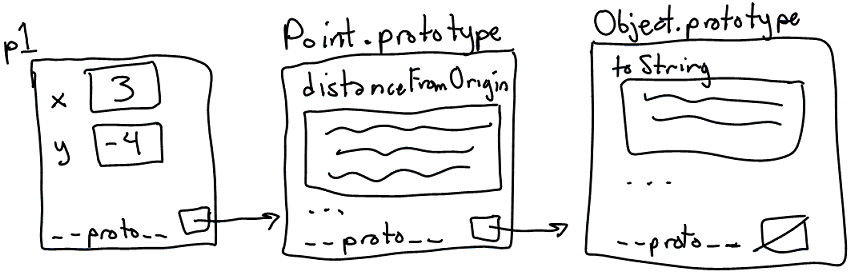# Web Programming Step by Step, 2nd Edition

## Lecture XX: Object-Oriented JavaScript

Except where otherwise noted, the contents of this document are Copyright 2012 Marty Stepp, Jessica Miller, and Victoria Kirst. All rights reserved. Any redistribution, reproduction, transmission, or storage of part or all of the contents in any form is prohibited without the author's expressed written permission.

# Lecture Outline

• Motivation for objects
• Creating custom objects
• Object prototypes and "classes"
• Pseudo-inheritance using prototypes
• The Prototype framework's features for classes and inheritance

# Why use classes and objects?• small programs are easily written without objects
• JavaScript treats functions as first-class citizens
• larger programs become cluttered with disorganized functions
• objects group related data and behavior
• helps manage size and complexity, promotes code reuse
• You have already used many types of JavaScript objects
• Strings, arrays, HTML / XML DOM nodes
• Prototype `Ajax.Request`, Scriptaculous `Effect` / `Sortable` / `Draggable`

# Creating a new anonymous object

```var name = {
fieldName: value,
...
fieldName: value
};
```
```var pt = {
x: 4,
y: 3
};
alert(pt.x + ", " + pt.y);
```
• in JavaScript, you can create a new object without creating a class
• the above is like a `Point` object; it has fields named `x` and `y`
• the object does not belong to any class; it is the only one of its kind
• `typeof(pt) === "object"`

```new Ajax.Request("http://example.com/app.php", {
method: "get",            // an object with a field named method (String)
onSuccess: ajaxSuccess    // and a method named onSuccess
});

duration: 2.0,            // an object with 3 fields named:
from: 1.0,                // duration, from, and to (Number)
to: 0.5
});
```
• the parameters in `{}` passed to Prototype/Scriptaculous were actually anonymous objects

# Objects that have behavior (functions/methods)

```var name = {
...
methodName: function(parameters) {
statements;
}
};
```
```var pt = {
x: 4,  y: 3,
distanceFromOrigin: function() {
return Math.sqrt(this.x * this.x + this.y * this.y);
}
};

```
• like in Java, objects' methods run "inside" that object
• inside an object's method, the object refers to itself as `this`
• unlike in Java, the `this` keyword is mandatory in JS

# A poor attempt at a "constructor"

What if we want to create an entire new class, not just one object?

• JavaScript, unlike Java, does NOT have classes
• we could emulate the functionality of a constructor with a function:
```// Creates and returns a new Point object. (This is bad code.)
function constructPoint(xValue, yValue) {
var pt = {
x: xValue,  y: yValue,
distanceFromOrigin: function() {
return Math.sqrt(this.x * this.x + this.y * this.y;
}
};
return pt;
}
```
```var p = constructPoint(4, -3);
```
• the above code is ugly and doesn't match the `new` syntax we're used to

# Constructor functions

```// Constructs and returns a new Point object.
function Point(xValue, yValue) {
this.x = xValue;
this.y = yValue;
this.distanceFromOrigin = function() {
return Math.sqrt(this.x * this.x + this.y * this.y);
};
}
```
```var p = new Point(4, -3);
```
• a constructor is just a normal function
• when any function called with `new`, JavaScript does the following:
• creates a new empty anonymous object and uses it as `this` within the function
• implicitly returns the new object at the end of the function
• what happens if our "constructor" is called as a normal function, without `new`?
```var p = Point(4, -3);
```

# Problems with our constructor

```// Constructs and returns a new Point object.
function Point(xValue, yValue) {
this.x = xValue;
this.y = yValue;
this.distanceFromOrigin = function() {
return Math.sqrt(this.x * this.x + this.y * this.y);
};
}
```
• ugly syntax; every method must be declared inside the constructor
• (subtle) replicates the methods in every object (wasteful)
• every `Point` object has its own entire copy of the `distanceFromOrigin` code• prototype: an ancestor of a JavaScript object
• like a "super-object" instead of a superclass
• a parent at the object level rather than at the class level
• not to be confused with Prototype framework
• every object contains a reference to its prototype
• the default is `Object.prototype`
• strings use `String.prototype`, etc.
• a prototype can have a prototype, and so on
• an object "inherits" all methods/data from its prototype(s)
• it doesn't have to make a copy of them, which saves memory
• prototypes allow JavaScript to mimic classes and inheritance

# An object's prototype chain• when you try to look up a property or method in an object, JavaScript:
1. Sees if the object itself contains that property/method.
2. If not, recursively checks the object's prototype to see if it has the property/method.
3. Continues up the "prototype chain" until it finds the property/method or gives up with `undefined`.

# Constructors and prototypes

```// also causes Point.prototype to become defined
function Point(xValue, yValue) {
...
}
```
• every constructor also has an associated prototype object
• example: when we define our `Point` constructor, that creates a `Point.prototype`
• initially this object has nothing in it
• every object you construct will use the constructor's prototype object as its prototype
• example: every constructed `Point` object will use `Point.prototype`
• (revised) when any function called with `new`, JavaScript does the following:
• creates a new empty anonymous object
• attaches the function's prototype object to the new object as its prototype
• runs the constructor's code, using the new object as `this`
• implicitly returns the new object at the end of the function

# Modifying a prototype

```// adding a method to the prototype
className.prototype.methodName = function(parameters) {
statements;
}
```
```Point.prototype.distanceFromOrigin = function() {
return Math.sqrt(this.x * this.x + this.y * this.y);
};
```
• adding a method/field to a prototype will give it to all objects using that prototype
• better than manually adding each method to each object (copying the method N times)
• we generally put only methods and constant data (not fields!) in a prototype object
• what would happen if we put the `x` and `y` fields in `Point.prototype`?
• Exercise: Add `distance` and `toString` methods.

# `Point` prototype methods

```// Computes the distance between this point and the given point p.
Point.prototype.distance = function(p) {
var dx = this.x - p.x;
var dy = this.y - p.y;
return Math.sqrt(dx * dx + dy * dy);
};

// Returns a text representation of this object, such as "(3, -4)".
Point.prototype.toString = function() {
return "(" + this.x + ", " + this.y + ")";
};
```
• our `Point` code could be saved into a file `Point.js`
• the `toString` method works similarly as in Java

# Modifying built-in prototypes

```// add a 'contains' method to all String objects
String.prototype.contains = function(text) {
return this.indexOf(text) >= 0;
};

// add a 'lightUp' method to all HTML DOM element objects
HTMLElement.prototype.lightUp = function() {
this.style.backgroundColor = "yellow";
this.style.fontWeight = "bold";
};
```
• ANY prototype can be modified, including those of existing types
• Prototype and other libraries do this
• not quite the same as adding something to a single object
• Exercise: Add a `reverse` method to strings.
• Exercise: Add a `shuffle` method to arrays.

# Practice problem: Favorite Movies• Add JavaScript to `moviegallery.html` to list your favorite movies.
• Create a new type called `Movie`:
• `Movie(title, genre, rating)` - constructor.
• `createHTML()` - Creates and returns an HTML DOM object representing a `div` holding the HTML for this movie.
```<div class="movie">
<button class="delete">X</button>
<p class="title">Indiana Jones 4</p>
<p class="rating">3/5</p>
</div>
```
• `deleteMe()` - Removes the movie from the page.

# Pseudo-inheritance with prototypes

```function SuperClassName(parameters) {   // "superclass" constructor
...
};
```
```function SubClassName(parameters) {     // "subclass" constructor
...
};
```
```SubClassName.prototype = new SuperClassName(parameters);   // connect them
```

• to make a "subclass", tell its constructor to use a "superclass" object as its prototype
• why not just write it this way?
```SubClassName.prototype = SuperClassName.prototype;   // connect them
```

# Pseudo-inheritance example

```// Constructor for Point3D "class"
function Point3D(x, y, z) {
this.x = x;
this.y = y;
this.z = z;
};

Point3D.prototype = new Point(0, 0);   // set as "subclass" of Point

// override distanceFromOrigin method
Point3D.prototype.distanceFromOrigin = function() {
return Math.sqrt(this.x * this.x + this.y * this.y + this.z * this.z);
};
```
• mostly works fine, but there no equivalent of the `super` keyword
• no built-in way to call an overridden method
• no easy way to call the superclass's constructor

# Classes and prototypes

• limitations of prototype-based code:
• unfamiliar / confusing to many programmers
• somewhat unpleasant syntax
• difficult to get inheritance-like semantics (subclassing, overriding methods)

# Creating a class

```className = Class.create({
// constructor
initialize : function(parameters) {
this.fieldName = value;
...
},

methodName : function(parameters) {
statements;
},
...
});
```
• constructor is written as a special `initialize` function

# `Class.create` example

```Point = Class.create({
// Constructs a new Point object at the given initial coordinates.
initialize: function(initialX, initialY) {
this.x = initialX;
this.y = initialY;
},

// Computes the distance between this Point and the given Point p.
distance: function(p) {
var dx = this.x - p.x;
var dy = this.y - p.y;
return Math.sqrt(dx * dx + dy * dy);
},

// Returns a text representation of this Point object.
toString: function() {
return "(" + this.x + ", " + this.y + ")";
}
});
```

# Inheritance

```className = Class.create(superclass, {
...
});
```
```// Points that use "Manhattan" (non-diagonal) distances.
ManhattanPoint = Class.create(Point, {
// Computes the Manhattan distance between this Point and p.
// Overrides the distance method from Point.
distance: function(p) {
var dx = Math.abs(this.x - p.x);
var dy = Math.abs(this.y - p.y);
return dx + dy;
},

// Computes this point's Manhattan Distance from the origin.
distanceFromOrigin: function() {
return this.x + this.y;
}
});
```

# Referring to superclass: `\$super`

```name: function(\$super, parameters) {
statements;
}
```
```ManhattanPoint3D = Class.create(ManhattanPoint, {
initialize: function(\$super, initialX, initialY, initialZ) {
\$super(initialX, initialY);   // call Point constructor
this.z = initialZ;
},

// Returns 3D "Manhattan Distance" from p.
distance: function(\$super, p) {
var dz = Math.abs(this.z - p.z);
return \$super(p) + dz;
},
});
```
• can refer to superclass's overridden method as `\$super` in code

# Practice problem: Fancy movies• Modify the movie gallery code from the last practice problem so that it uses Prototype's `Class.create` method to make the `Movie` type.
• Once this is done, add a new subtype `FancyMovie` that displays its movie ratings as star images rather than just showing a number on the page.